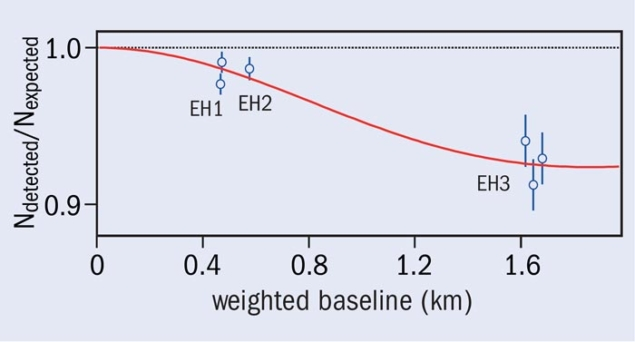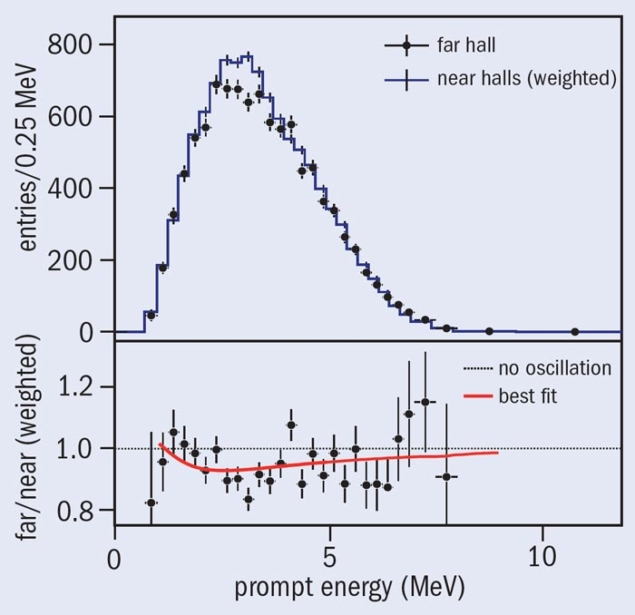# Daya Bay collaboration observes a new kind of neutrino oscillation

27 April 2012Fig. 1. Ratio of the detected number of electron-antineutrinos versus the number expected, assuming no oscillation in each detector. The expected signal has been corrected with the best-fit normalization parameter. The flux-weighted average baselines were computed using the reactor and survey data. The red curve is the oscillation-survival probability, with the best-fit value of sin22θ13 = 0.092. The left and right data points of the far hall are displaced by –30 and +30 m for visual clarity.

The Daya Bay reactor antineutrino experiment has observed the disappearance of electron-antineutrinos at a distance of about 2 km from the reactors. As briefly reported earlier, this provides strong evidence for a new kind of neutrino oscillation through a nonzero neutrino-mixing angle, θ13.

There has been good evidence for more than a decade that the electron-neutrino, muon-neutrino and tau-neutrino can morph into one another. This phenomenon of neutrino oscillation is a consequence of mixing between the three flavours of neutrinos, and oscillations between three neutrinos are described with three mixing angles, two mass-squared differences and one CP-violating phase. Two of the mixing angles, θ12 and θ23, have been measured to good precision but the third mixing angle, θ13, was poorly known.

A decade ago, the CHOOZ experiment set a limit of sin213 < 0.17. However, newer analyses of the measurements with solar neutrinos and by the KamLAND experiment – as well as data from the T2K, MINOS and Double Chooz experiments – hinted that θ13 could be larger than zero. On 8 March, based on an exposure of 43,000 tonne-GWth-days, the Daya Bay collaboration reported the result of their measurement, sin213 = 0.092 ± 0.016 (stat.) ± 0.005 (syst.), concluding that θ13 is significantly different from zero.

The Daya Bay experiment is located at the Daya Bay Nuclear Power Complex in China, 55 km northeast of Hong Kong. About 3.6 × 1021 low-energy electron-antineutrinos per second are produced by three pairs of nuclear reactors with a combined maximum thermal-power of 17.4 GWth. Three underground experimental halls connected by horizontal tunnels will eventually house eight antineutrino detectors (two in each near hall and four in the far site).

In each hall, the antineutrino detectors are submerged in a water pool that is partitioned optically into two zones. These two water-Cherenkov detectors tag cosmic-ray muons, which can generate background that mimics antineutrino interactions. The water also shields the detectors from ambient radiation that can generate background. The experiment identified electron-antineutrinos via the inverse beta-decay reaction νe + p → e+ + n, with 20 tonnes of 0.1% gadolinium-doped liquid scintillator in each antineutrino detector.

The data used for these first results were obtained with six antineutrino detectors – three deployed in the far hall, two in one of the near halls and one in the other near hall. When the number of detected electron-antineutrino events at the far site was compared with the expected number derived from the measurements in the near sites, a ratio of 0.940 ± 0.011 (stat.) ±0.004 (syst.) was found, indicating neutrino oscillation through θ13. Using the total number of detected events yielded a value of sin213 that was 5.2 σ from zero.

Figure 1 shows the disappearance of reactor electron-antineutrinos as a function of flux-weighted distance. Further evidence for this new kind of neutrino oscillation comes from the comparison of the observed and predicted energy spectra of the electron-antineutrinos at the far site. As figure 2 shows, the spectral distortion as a function of the prompt (positron) energy is also consistent with oscillation corresponding to sin213 = 0.092.Fig. 2. Top: The measured prompt-energy spectrum at the far hall (sum of three antineutrino detectors) compared with the no-oscillation prediction from the measurements of the two near halls. The spectra were background subtracted. Uncertainties are statistical only. Bottom: The ratio of measured and predicted no-oscillation spectra. The red curve is the best-fit solution with sin22θ13 = 0.092 obtained from the rate-only analysis. The dashed line is the no-oscillation prediction.

Since the announcement of Daya Bay’s measurement of a nonzero value for θ13, the RENO collaboration has reported the observation of the disappearance of electron-antineutrinos by their experiment based in Korea. The value that they find for sin213 is consistent with the results from Daya Bay.

A nonzero θ13 is crucial for designing experiments to search for CP-violation in the neutrino sector. These next-generation experiments will explore whether neutrinos oscillate differently from antineutrinos and answer the question of whether neutrinos can explain why matter is predominant in the universe. Furthermore, knowing the value of θ13 helps to complete the determination of the neutrino-mixing matrix and constrain models beyond the current Standard Model.

• The Daya Bay collaboration consists of 230 collaborators from 38 institutions worldwide. The experiment is supported by the funding agencies of China, the Czech Republic, Hong Kong, Russia, Taiwan and the US. Daya Bay is currently one of the largest collaborative scientific projects between China and the US.